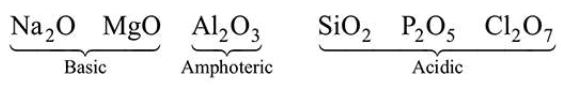# Three elements X, Y and Z are in the 3 period of the periodic table.`
Question:

Three elements $X, Y$ and $Z$ are in the $3^{\text {rd }}$ period of the periodic table. The oxides of X, Y and Z, respectively, are basic, amphoteric and acidic. The correct order of the atomic numbers of $X, Y$ and $Z$ is :

1. $\mathrm{Z}<\mathrm{Y}<\mathrm{X}$

2. $\mathrm{X}<\mathrm{Y}<\mathrm{Z}$

3. $X 4.$Y

Correct Option: , 2

Solution:

On moving left to right in a period, the acidic character of oxides increases.

$3^{\text {rd }}$ period element oxides.Acidic character $\propto$ Atomic No.

So, $\mathrm{X}$ have minimum atomic number while $\mathrm{Z}$ have maximum atomic number.

Thus, the correct order of the atomic number is

\$X